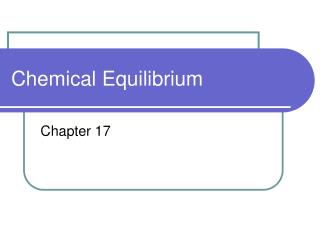DownloadDownload PresentationChemical Equilibrium

# Chemical Equilibrium

Download Presentation## Chemical Equilibrium

- - - - - - - - - - - - - - - - - - - - - - - - - - - E N D - - - - - - - - - - - - - - - - - - - - - - - - - - -
##### Presentation Transcript

1. Chemical Equilibrium Chapter 17

2. Review Given a chemical equation… A + B  C + D Which letters represent the products? Which letters represent the reactants? What is a rate? What is a concentration?

3. Reversible Reactions • A  B B  A Reversible reactions occur in both the forward and reverse directions CH4 + 2H2S CS2 + 4H2 Means that CH4 + H2S can form CS2 + H2 or CS2 + H2 can form CH4 + H2S Or A B

4. Equilibrium If A B When A  B B  A The reactions are at equilibrium when the forward and reverse reactions balance each other because they take place at equal rates r1 r1 = r2 equilibrium r2

5. Equilibrium • Amounts of products and reactants stays constant • Does not mean that there are equal amounts of products and reactants: [A] does not have to equal [B] • Catalysts speed both forward and backward rates, so they do not affect equilibrium concentrations • Law of chemical equilibrium: at a given temperature, reactant and product concentrations have a constant value

6. Equilibrium Constants For a reversible reaction at equilibrium aA + bB cC + dD Keq= [C]c x [D]d [A]a x [B]b Products over reactants Brackets indicate concentration If <1, more reactants than products If >1, more products than reactants Exponents same as coefficients in equation

7. Writing expressions for Homogeneous Equilibria • All in the same state of matter • Follow formula on previous slide Ex. Write the equilibrium constant expression for CH4(g) + 2H2S(g) CS2(g) + 4H2(g) Keq = [CS2][H2]4 [CH4 ][H2S]2

8. Writing expressions for Heterogeneous equilibria • Different states of matter • Because solids and liquids have a concentration that doesn’t change, you can omit them from the equilibrium expression Ex. What is the equilibrium constant expression for MgCO3(s) MgO(s) + CO2(g) ? [MgO][CO2] Keq= = [CO2] [MgCO3]

9. Calculating equilibrium constants • Plug in concentration data (experimental) into Keq expression Ex. At a certain temperature, the following concentrations are measured. Calculate Keq if CH4(g) + 2H2S(g) CS2(g) + 4H2(g) and [CH4 ]=0.5 mol/L [CS2]=0.5 mol/L [H2S]=0.7 mol/L [H2]=0.8 mol/L [CS2][H2]4 (0.5)(0.8)4 Keq= =0.84 [CH4 ][H2S]2 (0.5)(0.7)2

10. Calculating Keq • Calculate Keq for the following reaction: 2SO2 + O2 2SO3 If there are 0.1 mol , 0.2 mol, and 0.3 mol in a 1.0 L flask.

11. More on equilibrium constants • Keq should always equal the same thing for a given reaction at a given temperature • An infinite number of equilibrium positions: varies depending on initial concentration • Must be a closed system: no reactant or product can be added or escape • Temperature must remain constant • Dynamic: forward and reverse reactions don’t stop

12. Le Chatelier’s Principle • If a stress is applied to a system in dynamic equilibrium, the system changes to relieve the stress • 3 main stresses • Changing concentration of reactants or products • Changing the temperature • Changing the volume or pressure

13. Le Chatelier’s Principle • Concentration 2SO2 + O2 2SO3 Increasing the concentration of a reactant will shift the equilibrium so that more of it is used up Decreasing the concentration of a product will shift the equilibrium so that more of it is made Shifts reaction this way

14. Le Chatelier’s Principle • Pressure 2SO2 (g)+ O2 (g) 2SO3 (g) If pressure is increased (or volume decreased), it favors the side with fewer moles of gas If pressure is decreased (or volume increased), it favors the side with more moles of gas Shifts reaction this way If we increase the pressure… If we increase the volume…

15. Le Chatelier’s Principle • Temperature 2SO2 + O2 2SO3 + heat If heat is added, reaction will shift to favor the endothermic reaction (you can treat heat like a chemical) . If the forward reaction is exothermic and T increases, Keq decreases. Shifts reaction this way If we increase the temperature… If we decrease the temperature…

16. Using equilibrium constants • If you know an equation and Keq, you can calculate equilibrium concentrations. Ex. If 2ICl I2 + Cl2 Keq=0.110 at a particular temperature, [I2]=0.0330, and [Cl2]=0.220, what is the [ICl]? (0.033)(0.22) 0.110= [I2][Cl2] Keq= [ICl]2 [ICl]2 [ICl]=0.257 mol/L

17. Solubility Product Constants • Recall that compounds vary in solubility At saturation… AgCl(s) Ag+(aq) + Cl-(aq) So we can write a Keq for this process: Keq=[Ag+ ][Cl-] [AgCl] Ksp=[Ag+ ][Cl-] (multiply both sides by [AgCl]) Solubility product constant =1.7 x 10-5

18. Solubility Products Ksp=[Ag+ ][Cl-]= 1.7 x 10-5 What is the concentration of each ion at saturation? Since AgCl(s) Ag+(aq) + Cl-(aq) [Ag+]=[Cl-]=x So, x2 = 1.7 x 10-5

19. Predicting Precipitates and Calculating Ion Concentrations • To determine saturation you can do a trial solubility product (Qsp) and compare it to Ksp Ex. Will CaF2 precipitate if equal volumes 0.020 M Ca(NO3)2 and 0.0064 M NaF are mixed at 298 K? Ksp for CaF2=3.5 x 10-11 Qsp=[Ca2+ ][F-]2=(0.01)(0.0032)2=1.0 x10-7 Qsp > Ksp  Yes Note: concentrations are reduced by half because the volume of solution is now 2x as big

20. The Common Ion Effect • The solubility of a compound will decrease if you mix it with a solution with the same ion. • A precipitate will form • Ex: When mixing BaSO4 and NaSO4, BaSO4 will precipitate out

21. The Common Ion effect • What is the concentration of [Br-] if we add 0.020 mol AgNO3 to 1.00 L of saturated AgBr?